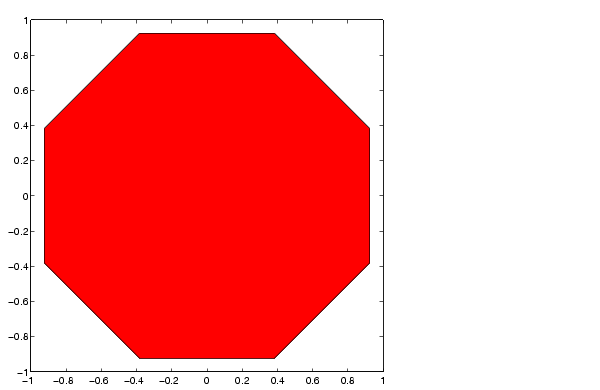MATLAB Function Referencefill

Filled two-dimensional polygons

Syntax

• fill(X,Y,C)
fill(X,Y,ColorSpec)
fill(X1,Y1,C1,X2,Y2,C2,...)
fill(...,'PropertyName',PropertyValue)
h = fill(...)

Description

The fill function creates colored polygons.

fill(X,Y,C) creates filled polygons from the data in X and Y with vertex color specified by C. C is a vector or matrix used as an index into the colormap. If C is a row vector, length(C) must equal size(X,2) and size(Y,2); if C is a column vector, length(C) must equal size(X,1) and size(Y,1). If necessary, fill closes the polygon by connecting the last vertex to the first.

fill(X,Y,ColorSpec) fills two-dimensional polygons specified by X and Y with the color specified by ColorSpec.

fill(X1,Y1,C1,X2,Y2,C2,...) specifies multiple two-dimensional filled areas.

fill(...,'PropertyName',PropertyValue) allows you to specify property names and values for a patch graphics object.

h = fill(...) returns a vector of handles to patch graphics objects, one handle per patch object.

Remarks

If X or Y is a matrix, and the other is a column vector with the same number of elements as rows in the matrix, fill replicates the column vector argument to produce a matrix of the required size. fill forms a vertex from corresponding elements in X and Y and creates one polygon from the data in each column.

The type of color shading depends on how you specify color in the argument list. If you specify color using ColorSpec, fill generates flat-shaded polygons by setting the patch object's FaceColor property to the corresponding RGB triple.

If you specify color using C, fill scales the elements of C by the values specified by the axes property CLim. After scaling C, C indexes the current colormap.

If C is a row vector, fill generates flat-shaded polygons where each element determines the color of the polygon defined by the respective column of the X and Y matrices. Each patch object's FaceColor property is set to 'flat'. Each row element becomes the CData property value for the nth patch object, where n is the corresponding column in X or Y.

If C is a column vector or a matrix, fill uses a linear interpolation of the vertex colors to generate polygons with interpolated colors. It sets the patch graphics object FaceColor property to 'interp' and the elements in one column become the CData property value for the respective patch object. If C is a column vector, fill replicates the column vector to produce the required sized matrix.

Examples

Create a red octagon.

• t = (1/16:1/8:1)'*2*pi;
x = sin(t);
y = cos(t);
fill(x,y,'r')
axis squarefilesep fill3